# AutoGluon 低调开源！性能超过人类调参师6个点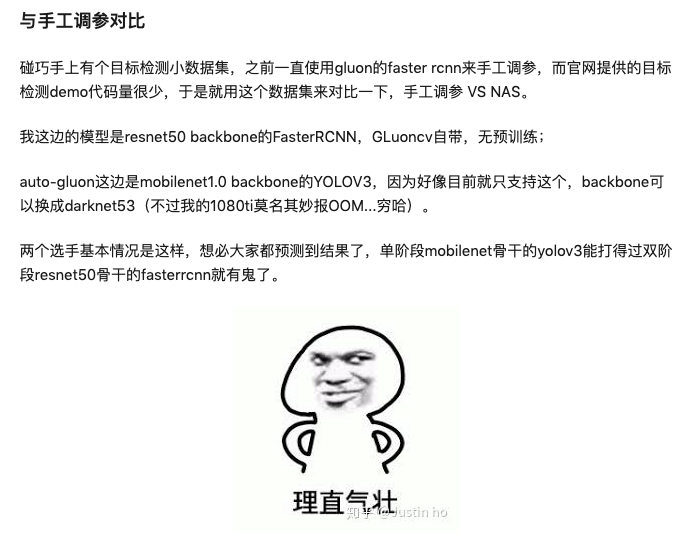「ResNet-50 backbone + FasterRCNN 骑脸怎么输嘛。」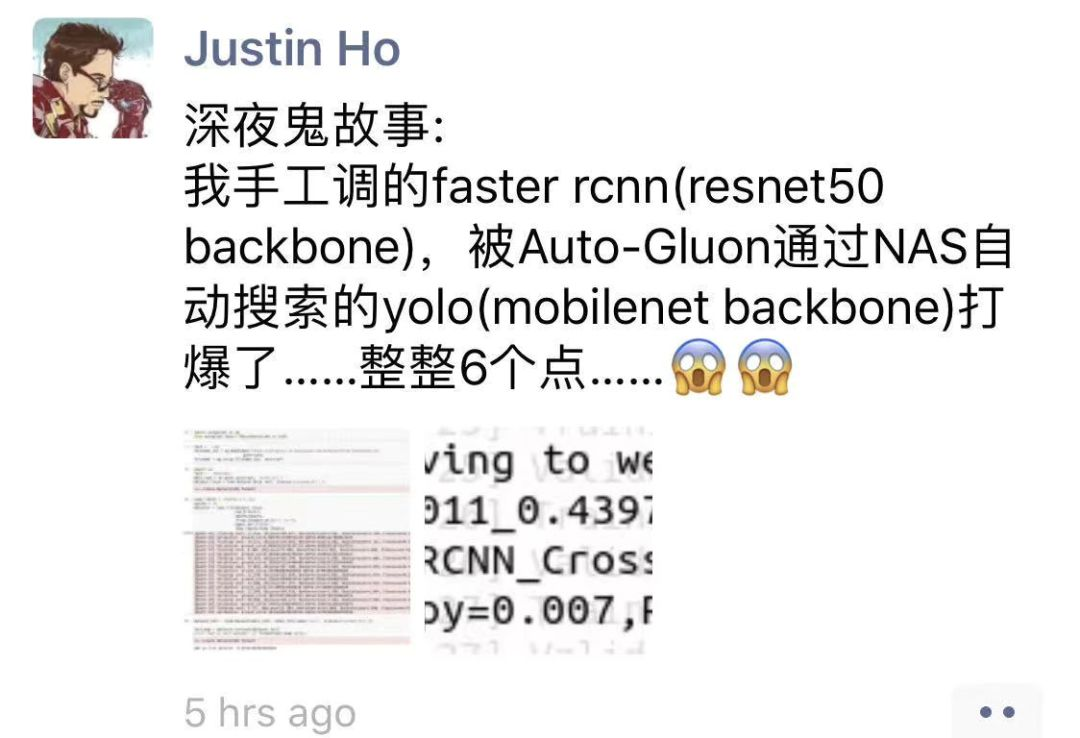AutoML 使用大概 15 倍于单次训练的代价，得到的结果可能比手调的要好。这个主要是对于 CV 而言，尤其是 detection 模型，预计 GluonCV 里面模型很快赢来一大波提升。

AutoGluon 取了一个巧，我们目前只支持 GluonCV 和 GluonNLP 里面的任务，和额外的 Tabular 数据（因为一个小哥之前有过经验）。所以我们可以把以前的很有经验东西放进去来减小搜参空间，从而提升速度。

AutoGluon：新特性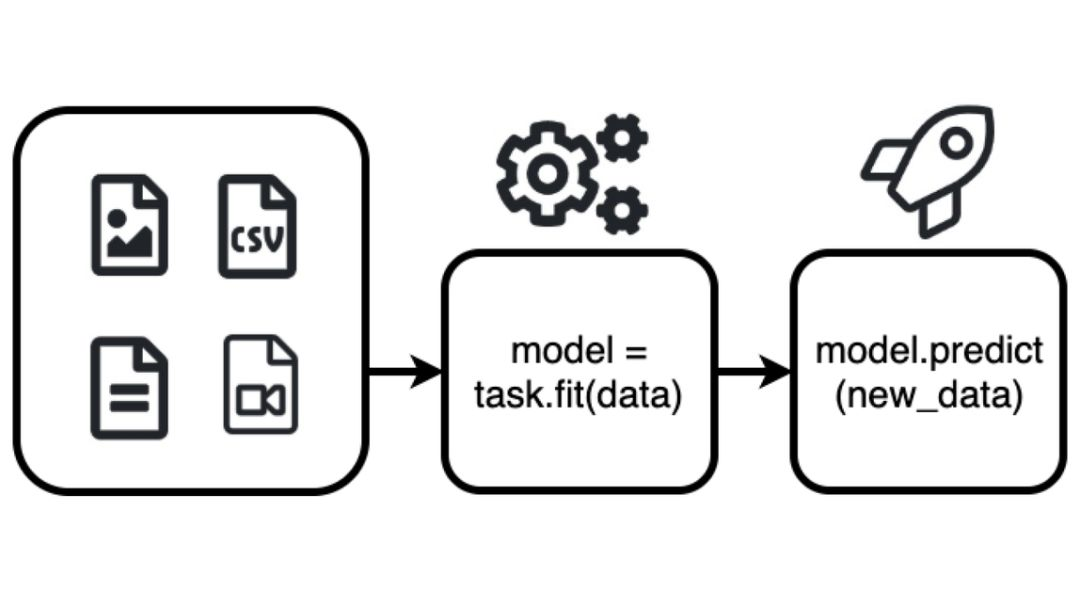图解 AutoGluon。

AutoGluon 支持易使用和易扩展的 AutoML，并专注于涵盖图像、文本或表格数据的深度学习和实际应用。AutoGluon 适用于机器学习初学者和专家，能够使他们：

• 通过几行代码即可快速地为数据构建深度学习原型方案；

• 利用自动超参数微调、模型选择/架构搜索和数据处理；

• 无需专家知识即可自动使用深度学习 SOTA 方法；

• 轻松地提升现有定制模型和数据管道，或者根据用例自定义 AutoGluon。

• 表格预测：基于数据表中一些列的值预测其他列的值；

• 图像分类：识别图像中的主要对象；

• 对象检测：借助图像中的边界框检测多个对象；

• 文本分类：基于文本内容做出预测。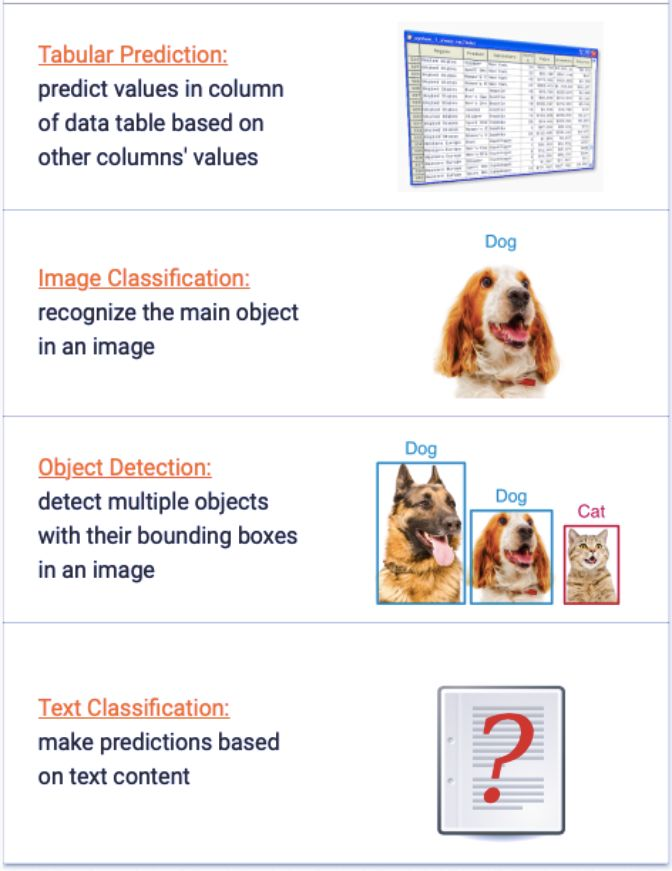import autogluon as ag
from autogluon import TabularPrediction as task


train_data = task.Dataset(file_path='https://autogluon.s3.amazonaws.com/datasets/Inc/train.csv')
train_data = train_data.head(500) # subsample 500 data points for faster demo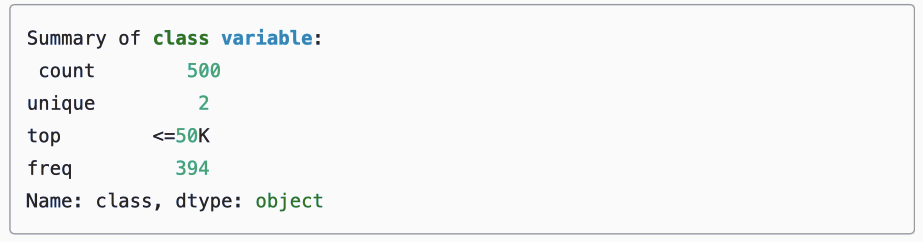dir = 'agModels-predictClass' # specifies folder where to store trained models


test_data = task.Dataset(file_path='https://autogluon.s3.amazonaws.com/datasets/Inc/test.csv')
y_test = test_data[label_column] # values to predict
test_data_nolab = test_data.drop(labels=[label_column],axis=1) # delete label column to prove we're not cheating


import autogluon as ag
from autogluon import ImageClassification as task


classifier = task.fit(dataset,epochs=10,ngpus_per_trial=1,verbose=False)


print('Top-1 val acc: %.3f' % classifier.results['best_reward'])


# skip this if training FashionMNIST on CPU.
if ag.get_gpu_count() > 0:
image = 'data/test/BabyShirt/BabyShirt_323.jpg'
ind, prob = classifier.predict(image)
print('The input picture is classified as [%s], with probability %.2f.' % (dataset.init().classes[ind.asscalar()], prob.asscalar()))


test_acc = classifier.evaluate(test_dataset)print('Top-1 test acc: %.3f' % test_acc)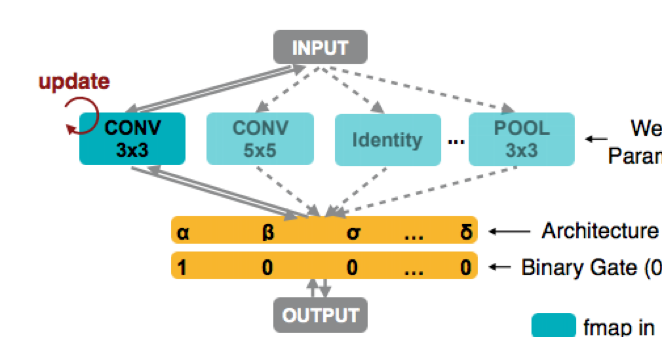ProxylessNAS 架构。

AutoGluon 也提供了相应的功能。

import autogluon as ag
import mxnet as mx
import mxnet.gluon.nn as nn


class Identity(mx.gluon.HybridBlock):
def hybrid_forward(self, F, x):
return x
class ConvBNReLU(mx.gluon.HybridBlock):
def __init__(self, in_channels, channels, kernel, stride):
super().__init__()
padding = (kernel - 1) // 2
self.conv = nn.Conv2D(channels, kernel, stride, padding, in_channels=in_channels)
self.bn = nn.BatchNorm(in_channels=channels)
self.relu = nn.Activation('relu')
def hybrid_forward(self, F, x):
return self.relu(self.bn(self.conv(x)))


from autogluon.contrib.enas import *

@enas_unit()
class ResUnit(mx.gluon.HybridBlock):
def __init__(self, in_channels, channels, hidden_channels, kernel, stride):
super().__init__()
self.conv1 = ConvBNReLU(in_channels, hidden_channels, kernel, stride)
self.conv2 = ConvBNReLU(hidden_channels, channels, kernel, 1)
if in_channels == channels and stride == 1:
self.shortcut = Identity()
else:
self.shortcut = nn.Conv2D(channels, 1, stride, in_channels=in_channels)

def hybrid_forward(self, F, x):
return self.conv2(self.conv1(x)) + self.shortcut(x)


mynet = ENAS_Sequential(
ResUnit(1, 8, hidden_channels=ag.space.Categorical(4, 8), kernel=ag.space.Categorical(3, 5), stride=2),
ResUnit(8, 8, hidden_channels=8, kernel=ag.space.Categorical(3, 5), stride=2),
ResUnit(8, 16, hidden_channels=8, kernel=ag.space.Categorical(3, 5), stride=2),
ResUnit(16, 16, hidden_channels=8, kernel=ag.space.Categorical(3, 5), stride=1, with_zero=True),
ResUnit(16, 16, hidden_channels=8, kernel=ag.space.Categorical(3, 5), stride=1, with_zero=True),
nn.GlobalAvgPool2D(), nn.Flatten(), nn.Activation('relu'), nn.Dense(10, in_units=16),)

mynet.initialize()
#mynet.graph


reward_fn = *lambda* metric, net: metric * ((net.avg_latency / net.latency) ** 0.1)


scheduler = ENAS_Scheduler(mynet, train_set='mnist',reward_fn=reward_fn, batch_size=128, num_gpus=1,warmup_epochs=0, epochs=1, controller_lr=3e-3,plot_frequency=10, update_arch_frequency=5)
scheduler.run()


AutoGluon 使用怎么样

https://github.com/awslabs/autogluon
https://www.amazon.science/amazons-autogluon-helps-developers-get-up-and-running-with-state-of-the-art-deep-learning-models-with-just-a-few-lines-of-code
https://venturebeat.com/2020/01/09/amazons-autogluon-produces-ai-models-with-as-little-as-three-lines-of-code/©️2019 CSDN 皮肤主题: 大白 设计师: CSDN官方博客